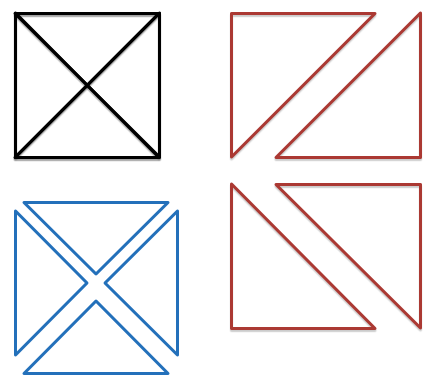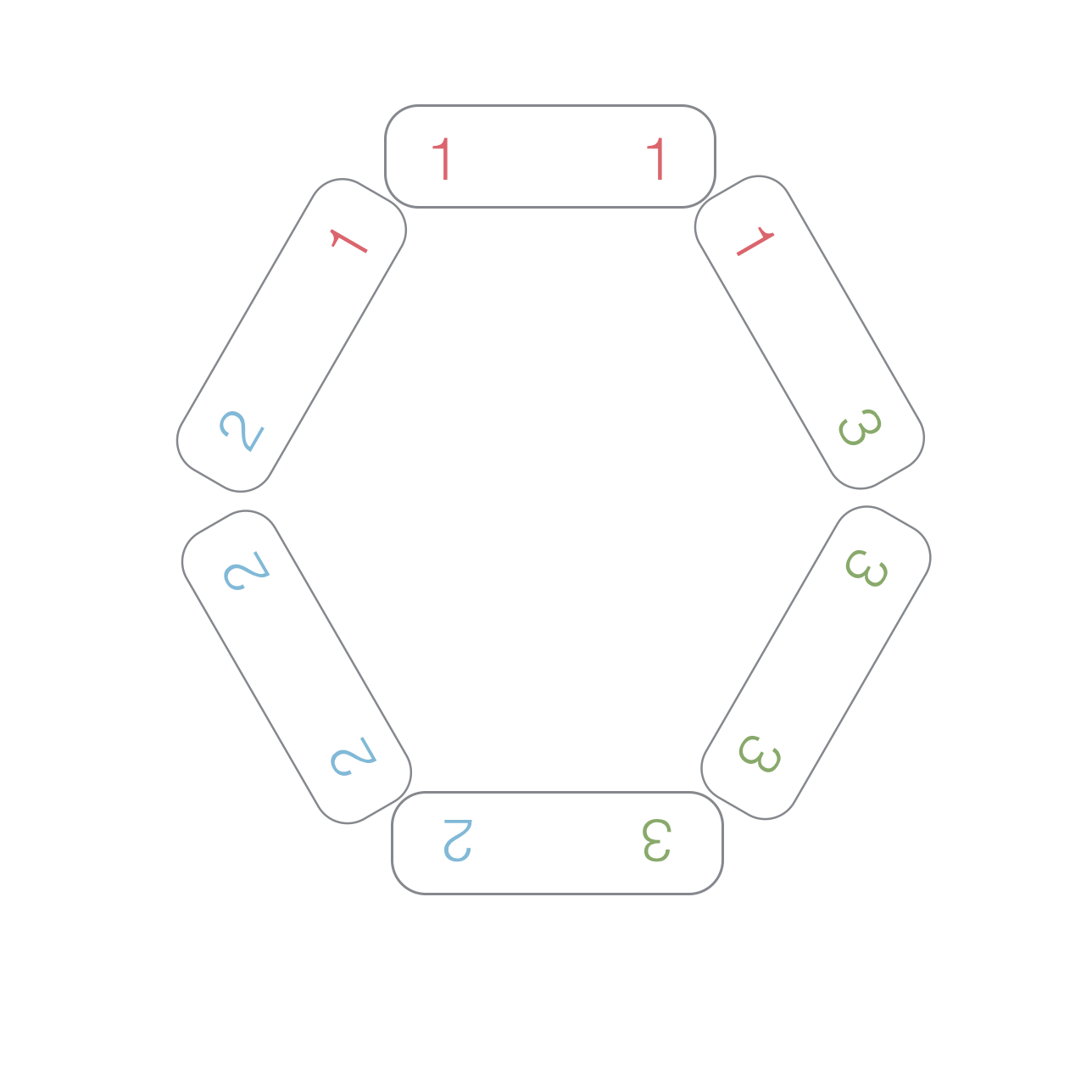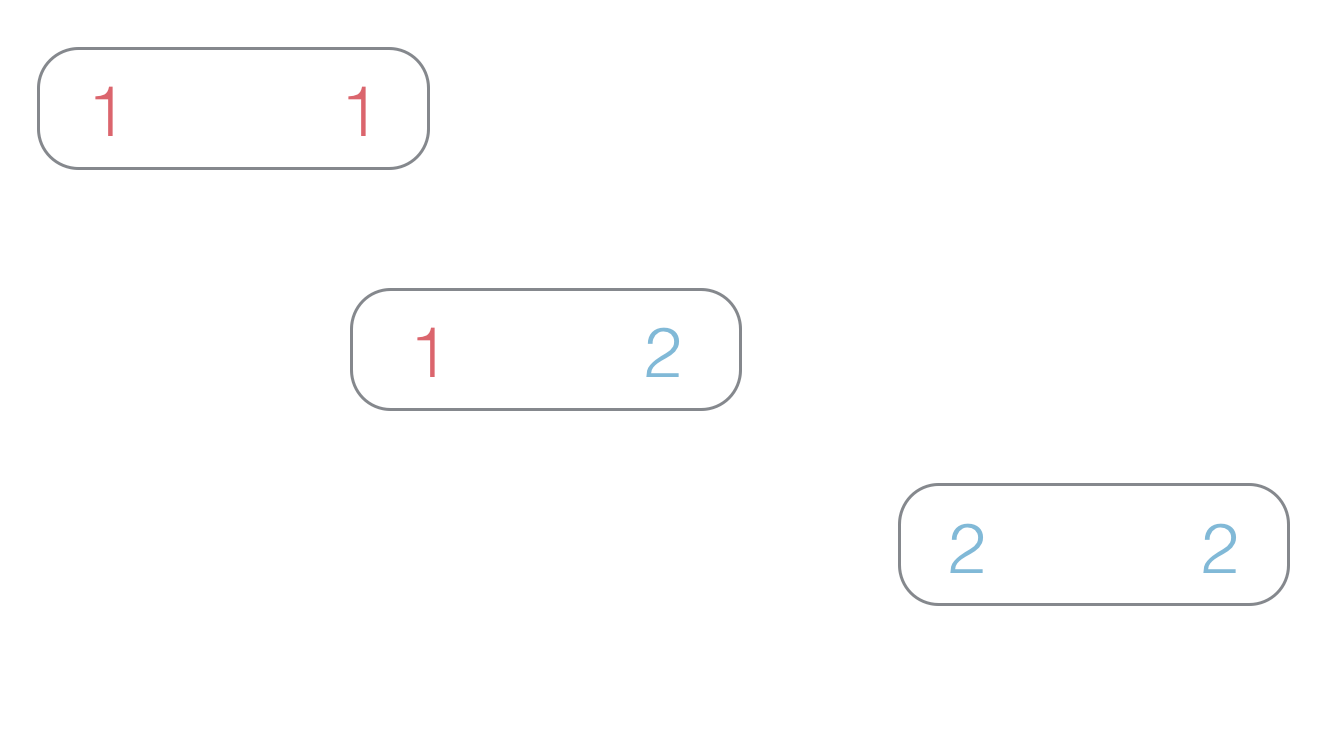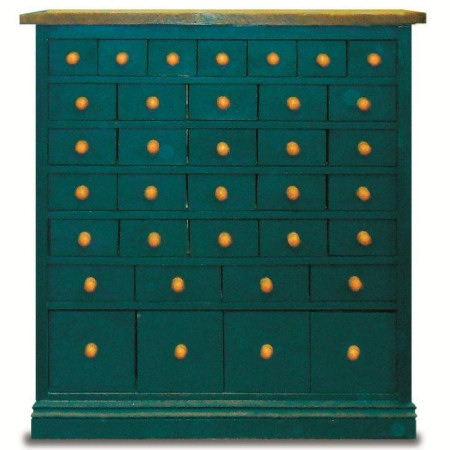Probability

# Graph Theory: Level 5 Challenges

For integers $n > 2$, let $G_n$ be a complete graph on $n$ vertices such that each vertex is labeled by a distinct number $1,2,3,\cdots,n$, and each edge is labeled by the sum of its endpoint labels. Let $f(G_n)$ be the minimum sum of edge labels in any path that touches every vertex in $G_n$ exactly once.

How many values of $n$ satisfy $f(G_n)\equiv 2013\pmod{n}?$A straight-line drawing of a graph $G$ is a drawing of $G$ on the plane such that all its edges are straight line segments.

The maximum number of triangles that can be found in a straight-line drawing of the complete graph $K_4$ is 8, as seen in the image. For $K_5$, there can be up to 35 triangles.

What is the maximum number of triangles that can be found in a straight-line drawing of the complete graph $K_{10}$?

The Chicago Art Museum's Renaissance display consists of four hallways bordered around a square courtyard. A single guard is assigned to patrol the four hallways. When the guard starts working, he begins in one of the corners and walks clockwise. When he arrives at a subsequent corner he flips two coins. If both coins are heads, he changes the direction he is walking. Otherwise, he continues in the same direction. Let $E$ be the expected number of lengths of hallway that he walks before he first returns to his starting corner. Let $p$ be the probability that he walks strictly more than $E$ lengths of hallway before returning to his starting corner. $p$ can be expressed as $\frac{a}{b}$ where $a$ and $b$ are coprime positive integers. What is the value of $a + b$?

Details and assumptions

The guard may walk in the same hallway more than one time. Each time he walks in it counts as one length of hallway.Consider a set of $\frac{ N (N+1) } { 2 }$ dominos which have faces of values 1 to N.
A ring of dominos is a closed loop of them, in which any 2 touching dominos display the same value.
Let $F(N)$ be the minimum numbers of rings that are needed to use up all of these dominos.

Find the last three digits of $\displaystyle \sum _{n = 1} ^{2016} F(n)$.

Inspiration

• As an explicit example, $F(3) = 1$ because we have the ring listed in the above image.
• For the purposes of this question, assume a single domino to be a ring. So $F(2) = 3$ (see image below) because there are three rings.There are 1000 prisoners and the warden decides to play the following game.

The prisoners are numbered 1 through 1000.
There is a room with 1000 boxes, numbered 1 through 1000.
There are 1000 pieces of paper, each numbered 1 through 1000.

The pieces of paper are randomly distributed one per box. (That is, paper #445 is just as likely to be in box #445, box #1, or any other box.)

Each prisoner will be taken in turn into the room with the boxes. He will open boxes of his choice one-by-one until either he finds the piece of paper with his own number on it or he has opened 500 boxes. Then he must leave and the room is reset. No communication between the prisoners is allowed once the game has begun.

If every prisoner finds the piece of paper with his or her number, then they will all be released. If a single prisoner fails to find his or her paper, then all of the prisoners will be locked up forever.

The prisoners are allowed to form a strategy before the game begins. What is the optimal strategy for the prisoners?

Approximately what is this strategy's probability of success?

[Note: there is both a cool graph-theoretic approach to this problem and also a group-theoretic approach. The end result of both is very simple and very beautiful. Have fun.]

×